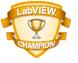# LabVIEW

cancel
Showing results for
Did you mean:

Highlighted

## Re:Newbie need help!Plots and compute average of 500 random number using Statistics

Hi,

I suppose to plots 500 random numbers on a waveform graph and compute the avearage of the random numbers using Statistics.

I have did the LabVIEW code for this problem but there is not any output show in the graph.

Should I put the statistic inside the loop instead?if yes,then there is wire connection problem for me to wire the waveform graph directly.

Thnx.

Rgds,

Vic

Message 1 of 5
(1,756 Views)

## Re: Re:Newbie need help!Plots and compute average of 500 random number using Statistics

None of that code is correct according to the desciption of your task. You are generating 5 numbers - not 500. The conversion to a DBL array and then autoindexing that into a 2D array is wrong. Just wire the value of the random number generator out of the for loop. This will geive you a 1D DBL array. Wire that to the graph. Also wire that to the Statistics function. Done. NO feedback.
Message 2 of 5
(1,741 Views)

## Re: Plots and compute average of 500 random number using Statistics

Hi,thanks for the hint.

Is my code correct now?

Message 3 of 5
(1,713 Views)

## Re: Plots and compute average of 500 random number using Statistics

Much better. I suspect that the Mean function on the Mathematics>Probablility & Statistics palette would have less overhead than the Express VI.

Also, it's a good idea to place a small wait in a while loop so that you do not starve the cpu.

Message 4 of 5
(1,709 Views)

## Re: Plots and compute average of 500 random number using Statistics

If you want to be able to see the results, you may want to modify your code as follows.

You can adjust the delay (ms) to your liking.  Or simply remove the While Loop as you had done in your other example.

You can also delete the error indicator.

I'm not sure why you are dividing 20 by the random number, but I left it as it was in your original code.Message Edited by Ray.R on 02-24-2010 01:13 PM
______________________________________________________________________Message 5 of 5
(1,694 Views)